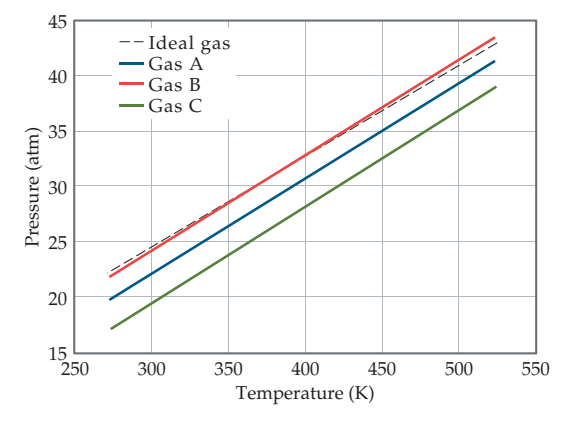×
Get Full Access to Chemistry: The Central Science - 14 Edition - Chapter 10 - Problem 10.12
Get Full Access to Chemistry: The Central Science - 14 Edition - Chapter 10 - Problem 10.12

×

# ?The graph below shows the change in pressure as the temperature increases for a 1-mol sample of a gas confined to a 1-L container. The four plots corrISBN: 9780134414232 1274

## Solution for problem 10.12 Chapter 10

Chemistry: The Central Science | 14th Edition

• Textbook Solutions
• 2901 Step-by-step solutions solved by professors and subject experts
• Get 24/7 help from StudySoup virtual teaching assistantsChemistry: The Central Science | 14th Edition

4 5 1 341 Reviews
18
0
Problem 10.12

The graph below shows the change in pressure as the temperature increases for a 1-mol sample of a gas confined to a 1-L container. The four plots correspond to an ideal gas and three real gases: $$\mathrm{CO}_{2}, \mathrm{~N}_{2}$$, and $$\mathrm{Cl}_{2}$$.

(a) At room temperature, all three real gases have a pressure less than the ideal gas. Which van der Waals constant, a or b, accounts for the influence intermolecular forces have in lowering the pressure of a real gas?

(b) Use the van der Waals constants in Table 10.3 to match the labels in the plot (A, B, and C) with the respective gases $$\left(\mathrm{CO}_{2}, \mathrm{~N}_{2}, \text { and } \mathrm{Cl}_{2}\right)$$. [Section 10.9]Text Transcription:

CO_2, N_2

Cl_2

(CO_2, N_2, and Cl_2)

Step-by-Step Solution:

Step 1 of 5) Another type of plastic is the elastomer, which is a material that exhibits rubbery or elastic behavior. When subjected to stretching or bending, an elastomer regains its original shape upon removal of the distorting force, if it has not been distorted beyond some elastic limit. Rubber is the most familiar example of an elastomer. 4 Recycling symbols. Most plastic containers manufactured today carry a recycling symbol indicating the type of polymer used to make the container and the polymer’s suitability for recycling.Some polymers, such as nylon and polyesters, both of which are thermosetting plastics, can be formed into fibers that, like hair, are very long relative to their cross-sectional area. These fibers can be woven into fabrics and cords and fashioned into clothing, tire cord, and other useful objects.

Step 2 of 2

## Discover and learn what students are asking

Calculus: Early Transcendental Functions : Basic Differentiation Rules and Rates of Change
?Finding a Derivative In Exercises 3–24, use the rules of differentiation to find the derivative of the function. $$y=x^{7}$$

Unlock Textbook Solution